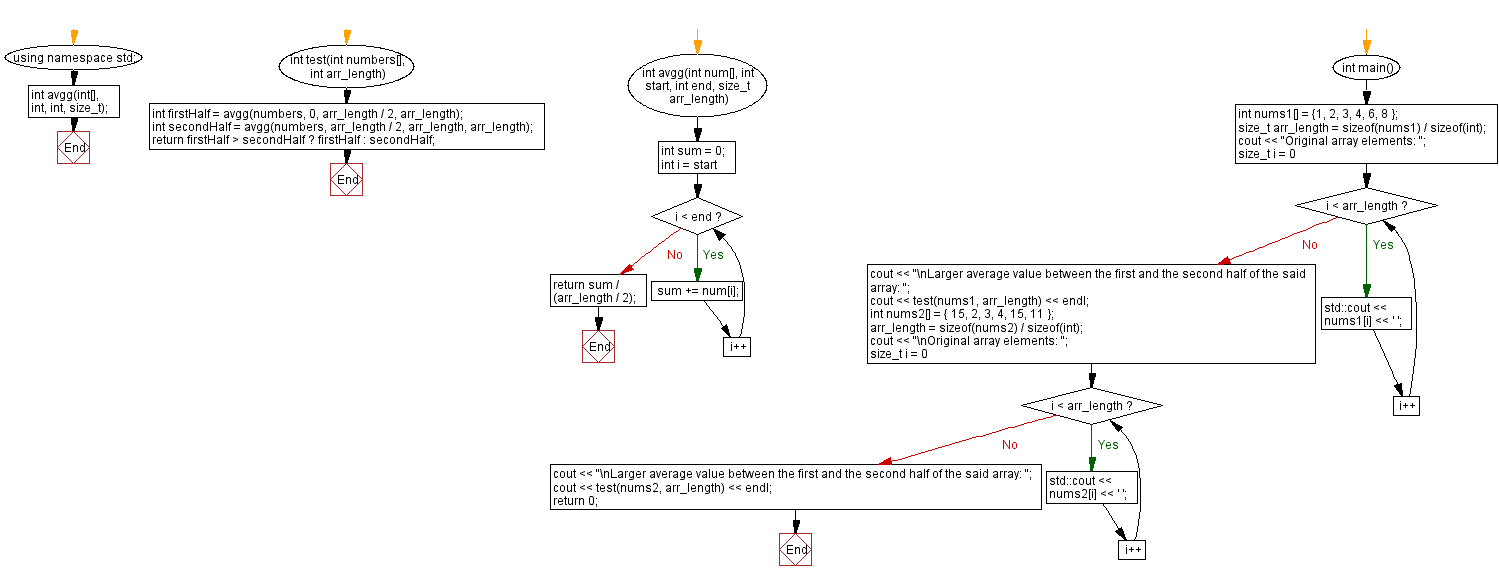﻿ C++ : Find the largest average value between the first and second halves of a given array of integers

# C++ Exercises: Find the largest average value between the first and second halves of a given array of integers

## C++ Basic Algorithm: Exercise-118 with Solution

Write a C++ program to find the largest average value between the first and second halves of a given array of integers. Ensure that the minimum length is at least 2. Assume that the second half begins at index (array length)/2.

Test Data:
({ 1, 2, 3, 4, 6, 8 }) -> 6
({ 15, 2, 3, 4, 15, 11 }) -> 10

Sample Solution:

C++ Code :

``````#include <iostream>

using namespace std;
int avgg(int[], int, int, size_t);
int test(int numbers[], int arr_length) {
int firstHalf = avgg(numbers, 0, arr_length / 2, arr_length);
int secondHalf = avgg(numbers, arr_length / 2, arr_length, arr_length);
return firstHalf > secondHalf ? firstHalf : secondHalf;
}
int avgg(int num[], int start, int end, size_t arr_length) {
int sum = 0;
for (int i = start; i < end; i++)
sum += num[i];
return sum / (arr_length / 2);
}
int main() {
int nums1[] = {1, 2, 3, 4, 6, 8 };
size_t arr_length = sizeof(nums1) / sizeof(int);
cout << "Original array elements: ";
for (size_t i = 0; i < arr_length; i++) {
std::cout << nums1[i] << ' ';
}
cout << "\nLarger average value between the first and the second half of the said array: ";
cout << test(nums1, arr_length) << endl;
int nums2[] = { 15, 2, 3, 4, 15, 11 };
arr_length = sizeof(nums2) / sizeof(int);
cout << "\nOriginal array elements: ";
for (size_t i = 0; i < arr_length; i++) {
std::cout << nums2[i] << ' ';
}
cout << "\nLarger average value between the first and the second half of the said array: ";
cout << test(nums2, arr_length) << endl;
return 0;
}
``````

Sample Output:

```Original array elements: 1 2 3 4 6 8
Larger average value between the first and the second half of the said array: 6

Original array elements: 15 2 3 4 15 11
Larger average value between the first and the second half of the said array: 10
```

Flowchart:C++ Code Editor:

What is the difficulty level of this exercise?

﻿

## C++ Programming: Tips of the Day

What is the usefulness of `enable_shared_from_this?

It enables you to get a valid shared_ptr instance to this, when all you have is this. Without it, you would have no way of getting a shared_ptr to this, unless you already had one as a member.

```class Y: public enable_shared_from_this
{
public:

shared_ptr f()
{
return shared_from_this();
}
}

int main()
{
shared_ptr p(new Y);
shared_ptr q = p->f();
assert(p == q);
assert(!(p < q || q < p)); // p and q must share ownership
}
```

The method f() returns a valid shared_ptr, even though it had no member instance. Note that you cannot simply do this:

```class Y: public enable_shared_from_this
{
public:

shared_ptr f()
{
return shared_ptr(this);
}
}
```

The shared pointer that this returned will have a different reference count from the "proper" one, and one of them will end up losing and holding a dangling reference when the object is deleted.

Ref : https://bit.ly/3pwVzzz